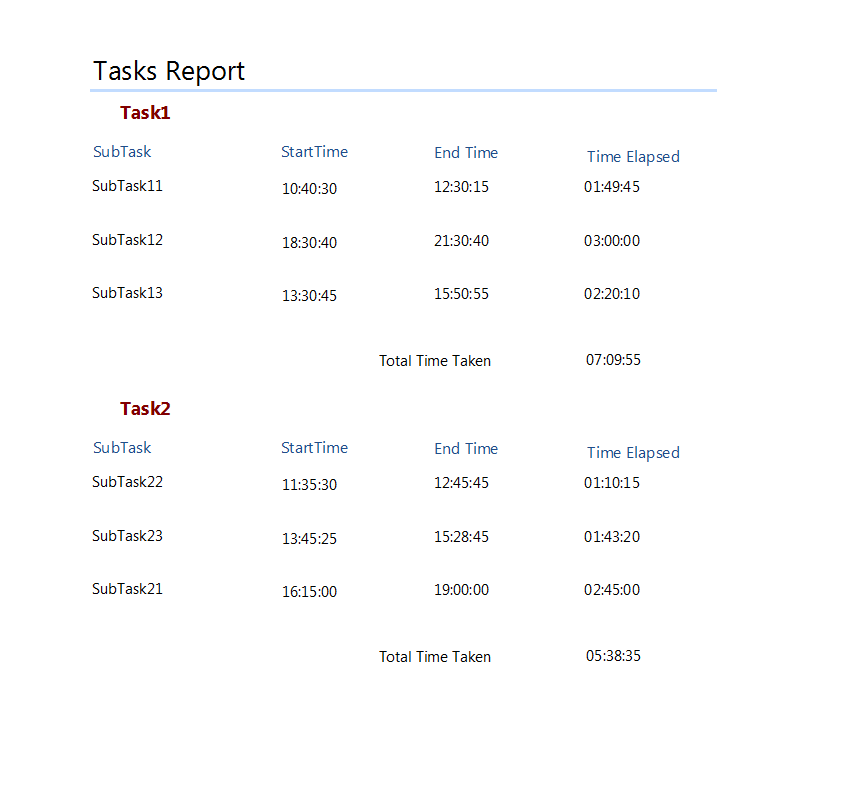﻿ Extending Time Functions in C1Report# Extending Time Functions in C1Report

VbScript does not support native arithmetic operations like Sum/Difference on Time /DateTime objects. This makes a situation a bit complex when applying direct VbScript functions (SUM/DIFF) on fields of type Time/DateTime in C1Report. Lets say you want a report with following layout:-So, how should we go about in these scenarios? We actually have to write custom functions and use them for calculating the required field values. The above defined report layout is very much self explanatory. 'Time Elapsed' field in the above report is a calculated one and the its value is calculated using the functions. Similarly, the 'Total Time' field is also a calculated one. The rest of the fields are from the bound datasource. Following are the simple steps :- 1) Define global variables 'diff_time', 'add_time' in OnOpen event of the report. 2) Write following function on the OnFormat event of the DetailSection:-

``````lSeconds = DateDiff("s", StartTime, EndTime)
lHrs = Int(lSeconds / 3600)
lMinutes = (Int(lSeconds / 60)) - (lHrs * 60)
lSeconds = Int(lSeconds Mod 60)
If lSeconds = 60 Then
lMinutes = lMinutes + 1
lSeconds = 0
End If
If lMinutes = 60 Then
lMinutes = 0
lHrs = lHrs + 1
End If
diff_time = lHrs & ":" & lMinutes & ":" & lSeconds
``````

The above function will be executed every time a row is formatted in a detail section and will calculate the value of the corresponding 'Time Elapsed' field. 3) Next is the vbscript which will sum up all the 'Time Elapsed' values in a group. You have to use this script in OnFormat event of the GroupFooter:-

``````lSeconds = add_time
lHrs = Int(lSeconds / 3600)
lMinutes = (Int(lSeconds / 60)) - (lHrs * 60)
lSeconds = Int(lSeconds Mod 60)
If lSeconds = 60 Then
lMinutes = lMinutes + 1
lSeconds = 0
End If
If lMinutes = 60 Then
lMinutes = 0
lHrs = lHrs + 1
End If
diff_time = lHrs & ":" & lMinutes & ":" & lSeconds
``````

4) Reset the 'add_time' value on the OnPrint event of the GroupSection so that the sum is not a running one. You can also use same functions in case you are creating the entire report in code.(Check attached sample.) DownloadSampleGrapeCity

GrapeCity Developer Tools